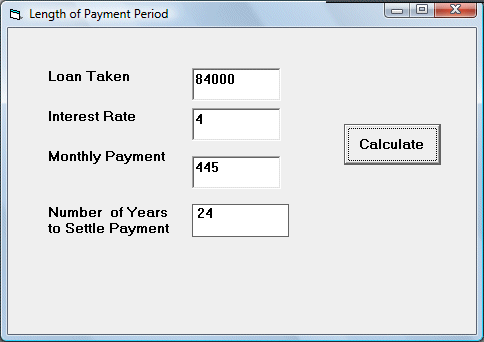# Payback Period Calculator

Currently people seem to face a lot of difficulties to secure a loan or have problem to pay back a loan. Still, we need to borrow money every now and then to acquire an asset or to pay for education fees. So, naturally we need to find out how long we can settle a loan for a certain amount of monthly payment at a certain interest rate. It is not easy to calculate such figure, fortunately VB comes to the rescue. There is a built-in function in VB to calculate the pay back period, it is known as Nper and the syntax is

Nper(Rate,Pmt,Pv,Fv,Due)

Rate=Interest Rate

Pmt=Amount of Periodic Payment

PV=Loan taken

FV=Future Value (set to 0 if loan is settled)

Due=set to 1 if payment at the beginning of the period

set to 0 if payment at the end of the period

### The Interface### The Code

Private Sub Command1_Click()
Dim payment, Loan, Int_Rate As Double
Dim Num_year As Single

payment = Val(Txt_Payment.Text)

Int_Rate = (Val(Txt_Rate.Text) / 100) / 12
Loan = Val(Txt_PV.Text)

Num_year = NPer(Int_Rate, payment, -Loan, 0, 0)/ 12

Lbl_Period.Caption = Str(Int(Num_year))
End Sub